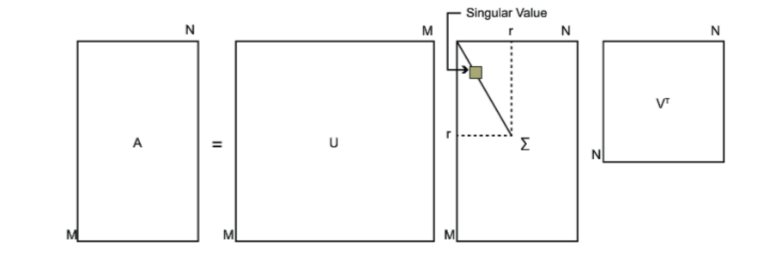$$Ax=\lambda x$$

• 解特征方程： $|A−λI_{n} |=0$
• 把每个特征值代入线性方程组$(A−λE)x=0$，求出基础解系。
• 求得的基础解系张成的特征子空间中所有向量均为对应特征值的全部特征向量。

$A=W∑W^{-1}$

## 奇异值分解

$$A = U\Sigma V^T$$

U和V都是酉矩阵，即满足$U^{T}U = I, V^{T}V = I$。$$(A^{T}A)v_{i} = \lambda_{i}v_{i}$$

$$(AA^T)u_i = \lambda_i u_i$$

U和V我们都求出来了，现在就剩下奇异值矩阵Σ没有求出了。由于Σ除了对角线上是奇异值其他位置都是0，那我们只需要求出每个奇异值σ就可以了。

$$A=U\Sigma V^T \Rightarrow AV=U\Sigma V^TV \Rightarrow AV=U\Sigma \Rightarrow Av_i = \sigma_i u_i \Rightarrow \sigma_i = Av_i / u_i$$

$$A=U\Sigma V^T \Rightarrow A^T=V\Sigma^T U^T \Rightarrow A^TA = V\Sigma^T U^TU\Sigma V^T = V\Sigma^2V^T$$

$U^TU=I, \Sigma^T\Sigma=\Sigma^2$。

$\sigma_i = Av_i / u_i$来计算奇异值，也可以通过求出$A^{T}A$的特征值取平方根来求奇异值。

Last modification：July 21st, 2019 at 08:18 pm
If you think my article is useful to you, please feel free to appreciate